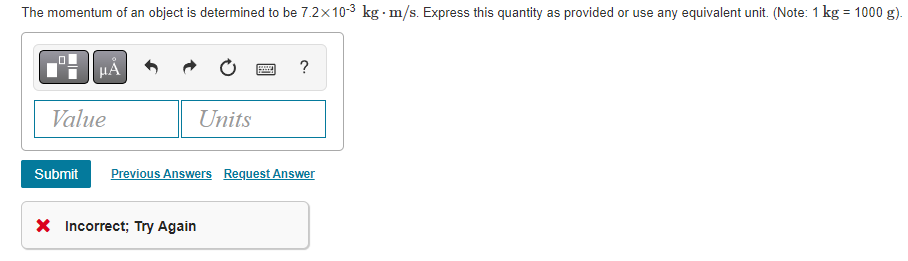# Question Solved1 AnswerTwo dimensional dynamics often involves solving for two unknown quantities in two separate equations describing the total force. The block in (Figure 1) has a mass m=10kgm=10kg and is being pulled by a force FF on a table with coefficient of static friction μs=0.3μs=0.3. Four forces act on it: The applied force FF (directed θ=30∘θ=30∘ above the horizontal). The force of gravity Fg=mgFg=mg (directly down, where g=9.8m/s2g=9.8m/s2). The normal force NN (directly up). The force of static friction fsfs (directly left, opposing any potential motion). If we want to find the size of the force necessary to just barely overcome static friction (in which case fs=μsNfs=μsN), we use the condition that the sum of the forces in both directions must be 0. Using some basic trigonometry, we can write this condition out for the forces in both the horizontal and vertical directions, respectively, as: Fcosθ−μsN=0Fcos⁡θ−μsN=0 Fsinθ+N−mg=0Fsin⁡θ+N−mg=0 In order to find the magnitude of force FF, we have to solve a system of two equations with both FF and the normal force NN unknown. Use the methods we have learned to find an expression for FF in terms of mm, gg, θθ, and μsμs (no NN). Express your answer in terms of mm, gg, θθ, and μsμs. F=? 1 kg=1000 g)Two dimensional dynamics often involves solving for two unknown quantities in two separate equations describing the total force. The block in (Figure 1) has a mass m=10kgm=10kg and is being pulled by a force FF on a table with coefficient of static friction μs=0.3μs=0.3. Four forces act on it:

• The applied force FF (directed θ=30∘θ=30∘ above the horizontal).
• The force of gravity Fg=mgFg=mg (directly down, where g=9.8m/s2g=9.8m/s2).
• The normal force NN (directly up).
• The force of static friction fsfs (directly left, opposing any potential motion).

If we want to find the size of the force necessary to just barely overcome static friction (in which case fs=μsNfs=μsN), we use the condition that the sum of the forces in both directions must be 0. Using some basic trigonometry, we can write this condition out for the forces in both the horizontal and vertical directions, respectively, as:

• Fcosθ−μsN=0Fcos⁡θ−μsN=0
• Fsinθ+N−mg=0Fsin⁡θ+N−mg=0

In order to find the magnitude of force FF, we have to solve a system of two equations with both FF and the normal force NN unknown. Use the methods we have learned to find an expression for FF in terms of mm, gg, θθ, and μsμs (no NN).

F=?Transcribed Image Text: 1 kg=1000 g)
More
Transcribed Image Text: 1 kg=1000 g)